# Damped Harmonic Oscillator

In a simple harmonic oscillator, no other force except the linear restoring force acts. As a result, the oscillator executes vibrations of constant amplitude and with a constant frequency these oscillations are called the free oscillations. The total mechanical energy (i.e., kinetic energy + potential energy) of the oscillator always remains conserved in such oscillations.

But in practice, free oscillations are not possible. This is because the medium in which the oscillator executes vibrations, exerts a frictional or viscous force on the oscillator. This force is called the damping force. This force acts in direction opposite to the direction of motion due to which the amplitude of vibration gradually decreases. Hence, the energy of oscillator also decreases. Such a vibrating system is called damped harmonic oscillator. For example, the simple pendulum executing oscillations in air or in any other medium, tuning fork, ballistic

galvanometer are the damped harmonic oscillators.

Thus, a particle executing damped harmonic motion in a medium (i.e., damped harmonic oscillator) is acted upon by two forces: ·

(i) Restoring force which is directly proportional to the displacement from a fixed point on its path and is in a direction opposite to the displacement, i.e., -Kx where K is froce constant and x is the displacement, is the displacement of the particle at any instant.

(ii) Damping force, which is proportional to the velocity of the particle and is in a direction opposite to motion, i.e., =r[dx/dt] , where r is a positive constant, which is called the damping coefficient.

If m is the mass of the particle, by Newton’s law, the total force acting on the particle= m-d2x dt2  where d2x/dt2 acceleration of the particle.

Thus the equation of motion of a damped harmonic oscillator will be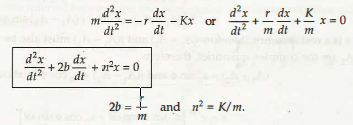.

It must be noted that unit of damping coefficient r is kg/ sec. Therefore, the unit of r/ m or 2b) is s-1. Thus the unit of m/r is same as that of the time. Hence the quantity m/r (or l/2b) is called the time constant or the relaxation time. It is represented by t (Tau). Relaxation time is defined as the time in which the energy of particle reduces to .1 I e (or 0.37) times its maximum initial energy.

Now, we are to find the solution of equation (1) for damped motion. Let the solution of equation (1) be x = Aeat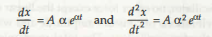Substituting these values in equation (1)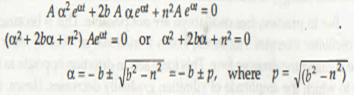Thus the two possible solutions of equation (1) are

x = A1 e( b + p)t and x = A2 e(-b-p) t

The general solution of equation (1) is

x = A1 e(b + p) t + A2 e(-b- p) t

=e-b1 [A1 eP1 + A 2e-P1]

Here, A1 and A2 are the constants whose values can be obtained from the initial condition of motion. There are following three cases possible:

1. When b < n, under damped.

2. When b > n, over damped.

3. When b = n, critically damped.

Case 1. Under-damped Case: If the damping is so small that b << n or r /2m<< √ Kim, then, p = √(b2- n2) = √- (n2 – b2) = √(n2- b2) or p = jw, where w = √(n2- b2).

Obviously, p is an imaginary quantity, but w is a real quantity.

Then from equation (2) X =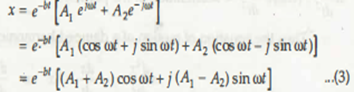Since x is a real quantity, therefore (A1 + A2) and j(A1 – A2)  must also be real  quantities. But A1 and A2 are the complex quantities, therefore

(A1 + A2) = a0 sin φ and  j(A1 – A2) = a0  cos  φ in above .equation (3)

x = e -bt [ a0 sin φ cos wt + a0 cos φ sin wt]

 x =ao e-bt sin (wt+φ)

This expression represents the damped harmonic motion where amplitude decreases exponentially with time. Hence, it is not a simple harmonic motion but it is an oscillatory motion, whose angular frequency is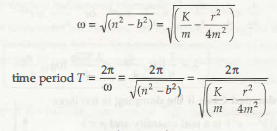The amplitude of motion is a = a0 e-bt = a0 e-1/2T

The displacement-time graph for the damped harmonic oscillations is shown in Figure .1 10. It is evident that the amplitude of motion decreases exponentially.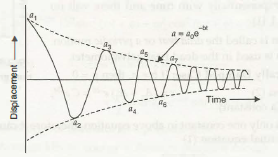FIGURE 1.10 Displacement-time graph for the damped harmonic oscillations

Logarithmic Decrement: The ratio of two successive amplitudes remains constant =( d)

and this ratio is called decrement i.e.,

a1/a2=a2/a3=a3/a4=…an/an-1=d(constant)

Since, the time interval between the two consecutive amplitudes on either side of mean position is T /2, therefore

Hence,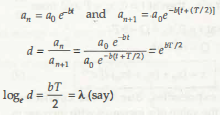or

Here, f.. is called the logarithmic decrement. Thus from equation (7)., d =eh

a1/a2 = a2/a3 = a3/a4… =an/an+1 = eh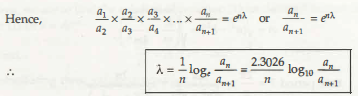Case 2. Over-damped Case: If the damping is too large that b > n, then p = √(b2- n2) is a real quantity and p <b.

Then from equation ( 4)  x=a1e–(b-p)t+a2e-(b+p)t

Since, p < b, hence’ both the quantities on RHS of above equation decrease exponentially with time and there will no oscillations (Figure 1.11).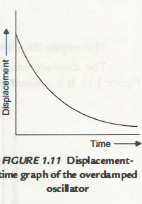Such a motion is called the dead beat or a periodic motion. This type of motion is used in the dead beat galvanometer.

Case 3. Critically Damped Case: If b = n, then p = 0

From equation (2)                  x = (A1 + A2) e-bt = C e-bt,

Where A1+A2 =c (a contant)

Since, there is only one constant in above equation, therefore it cannot be the solution of

second order differential equation (1)

Now if we consider that √(b2 – n2) =p a very small quantity, then

x = e-bt [ A1 ePt + A2 e-pt]

= e-bt [A1 (1 + pt + … ) + A2 (1- pt + … )]

Since, p is very small, therefore neglecting the terms p2 and p3, …

x = e-bt [(A1 +A2) + p (A1 – A2)t] = e-bt [P + Qt]

where, A1 + A2 = P and p[A1 + A2] = Q

If initially at t= 0, the displacement of particle is x= x0

and velocity v = dx/d t= vO’ then at t = 0, x0 = P and from dx/ dt

= Qe-bt – be-bt (P + Qt) at t = 0, v0 = Q- b P.

Hence, Q = v0 + bx0

Then,  x = [x0 + (v0 + bxo) t] e-bt                                                                     … (9)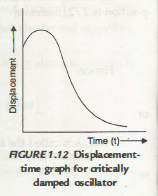In the above expression, due to the coefficient [x0 + (v0 + bx0) t], first the value of x increases with increase in t, but later on the exponential term e-bt becomes more pronounced due to which the value of x falls to zero in a small time interval and no oscillations occur.

1.7 .1 Power Dissipation in Damped Harmonic Oscillator

We have read that in damped oscillations, the mechanical energy of the particle continuously decreases due to the damping forces. As a result, the amplitude of motion also decreases with time. The displacement of particle executing damped harmonic motion at any instant tis

x =a0e-bt sin (wt+φ) from education

Velocity of the particle =  = dx/ dt = a0e-bt [(-b) sin (wt+ φ) + w cos (wt + φ)]

If m is the mass of the particle, the kinetic energy of the particle is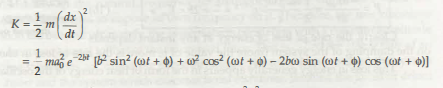And potential energy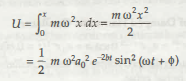Average total energy of oscillator in a periodic time

= Average kinetic energy + Average potential energy

Now, to evaluate the average kinetic energy and the average potential energy in one time period, we can assume that the amplitude of oscillation remains nearly unchanged i.e.,

e-2b1 remains nearly constant. Since the average value of sin2 (wt +φ)and cos2 (wt +φ)in 1/2 for one time period and average value of sin (wt + φ)cos (wt + φ) is zero over one time period, therefore in a period of time.

Average Kinetic energy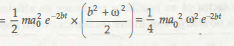And the average potential energy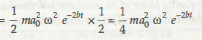Hence, the average total energy in one periodic time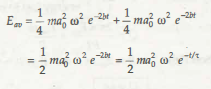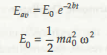It is clear from equation (15) that the average energy of system in damped oscillations decreases exponentially with time as shown in Figure 1.13.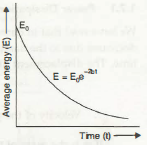The rate of loss of energy or the average power loss at any instant

Clearly, the rate of loss of energy at any instant depends average energy with time in damped oscillator on the damping of the system (more the value of b, more is the rate of loss in energy).

This loss in energy generally appears in the form of heat energy of the oscillating system.

1.7.2 Relaxation Time of Damped Harmonic Oscillator

If t = -r in equation a = a0 e-bt = a0 e-112-c then a= a0 e-112 = 0.6065 a0.

Hence, relaxation time in damped simple harmonic oscillator is that time in which its amplitude decreases to 0.6065 time its initial value.

In other way, from equation  (15)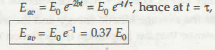Hence, the relaxation time in damped simple harmonic oscillator is that time in which its total energy reduces to 0.37 times its initial value.

1.7 .3 Quality Factor of Damped Harmonic Oscillator

The quality factor of an oscillator expresses its efficiency. It is represented by the letter Q. Since there is no energy loss in free oscillations of an ideal oscillator, its efficiency is maximum.

Definition: The quality factor of an oscillator is defined as the product of 2n with the ratio of the average energy stored in the oscillator at any instant to the loss in energy in one periodic time.

i.e., Quality factor   Q =2π x energy stored at any instant / energy lost in one second

But from equation (16)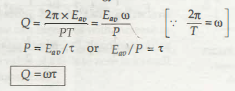Thus, quality factor is a dimensionless quantity. More the relaxation time of an oscillator, more is its quality factor.